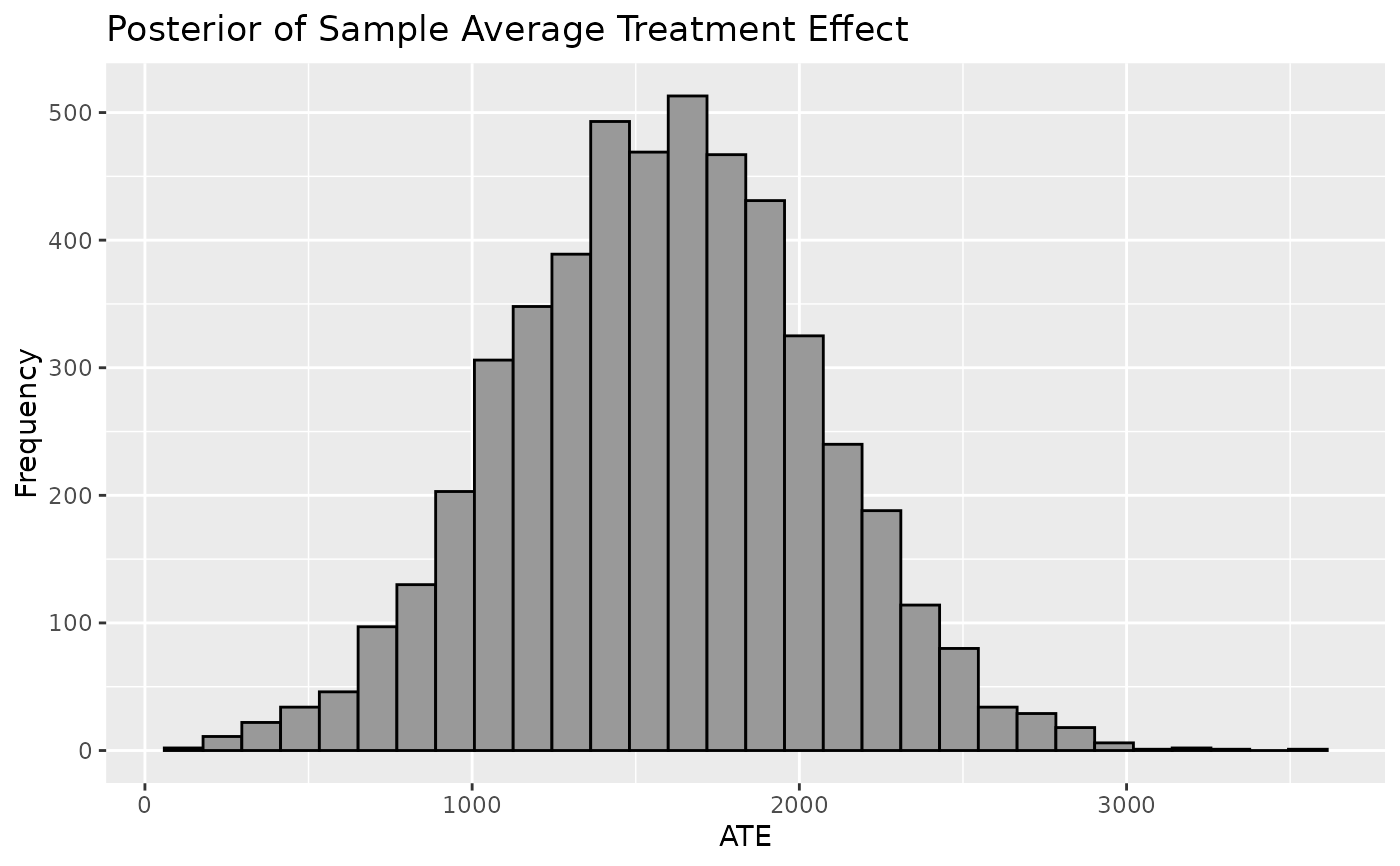Plot a histogram or density of the Sample Average Treatment Effect (SATE). The Sample Average Treatment Effect is derived from taking the difference of each individual's observed outcome and a predicted counterfactual outcome from a BART model averaged over the population. The mean of SATE will resemble means of CATE and PATE but will account for the least uncertainty.

## Usage

plot_SATE(
.model,
type = c("histogram", "density"),
ci_80 = FALSE,
ci_95 = FALSE,
reference = NULL,
.mean = FALSE,
.median = FALSE,
check_overlap = FALSE,
overlap_rule = c("none", "sd", "chisq")
)

## Arguments

.model

a model produced by bartCause::bartc()

type

histogram or density

ci_80

TRUE/FALSE. Show the 80% credible interval?

ci_95

TRUE/FALSE. Show the 95% credible interval?

reference

numeric. Show a vertical reference line at this x-axis value

.mean

TRUE/FALSE. Show the mean reference line

.median

TRUE/FALSE. Show the median reference line

check_overlap

TRUE/FALSE. Check if any overlap rules are applicable

overlap_rule

enter overlap rules to view how different bartCause removal rules would have influenced results. Only applicable if check_overlap is TRUE.

ggplot object

## Author

George Perrett, Joseph Marlo

## Examples

# \donttest{
data(lalonde)
confounders <- c('age', 'educ', 'black', 'hisp', 'married', 'nodegr')
model_results <- bartCause::bartc(
response = lalonde[['re78']],
treatment = lalonde[['treat']],
confounders = as.matrix(lalonde[, confounders]),
estimand = 'ate',
commonSup.rule = 'none'
)
#> fitting treatment model via method 'bart'
#> fitting response model via method 'bart'
plot_SATE(model_results)
#> stat_bin() using bins = 30. Pick better value with binwidth.# }